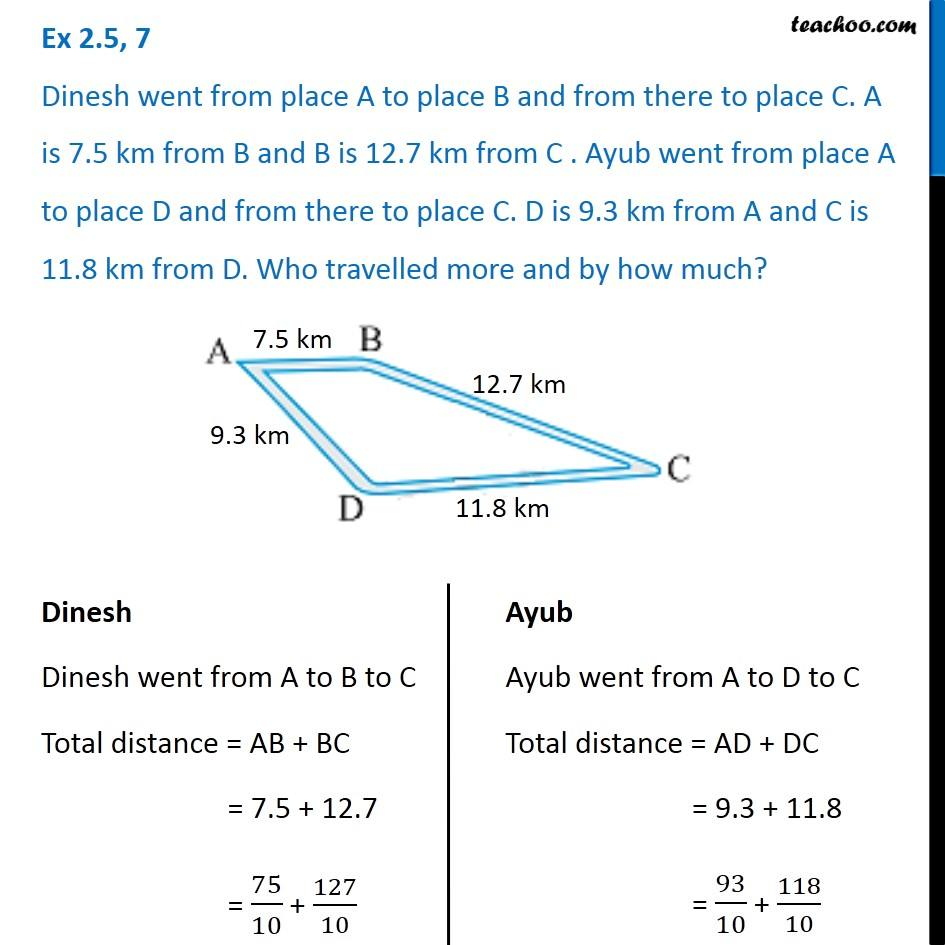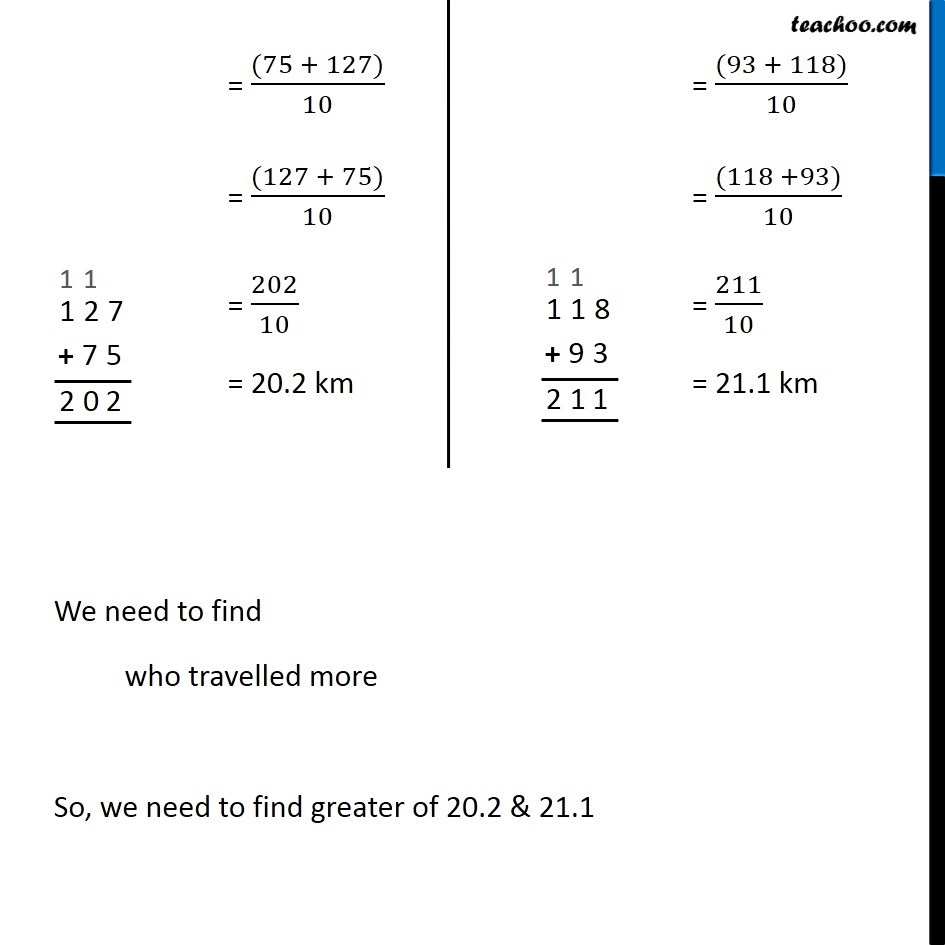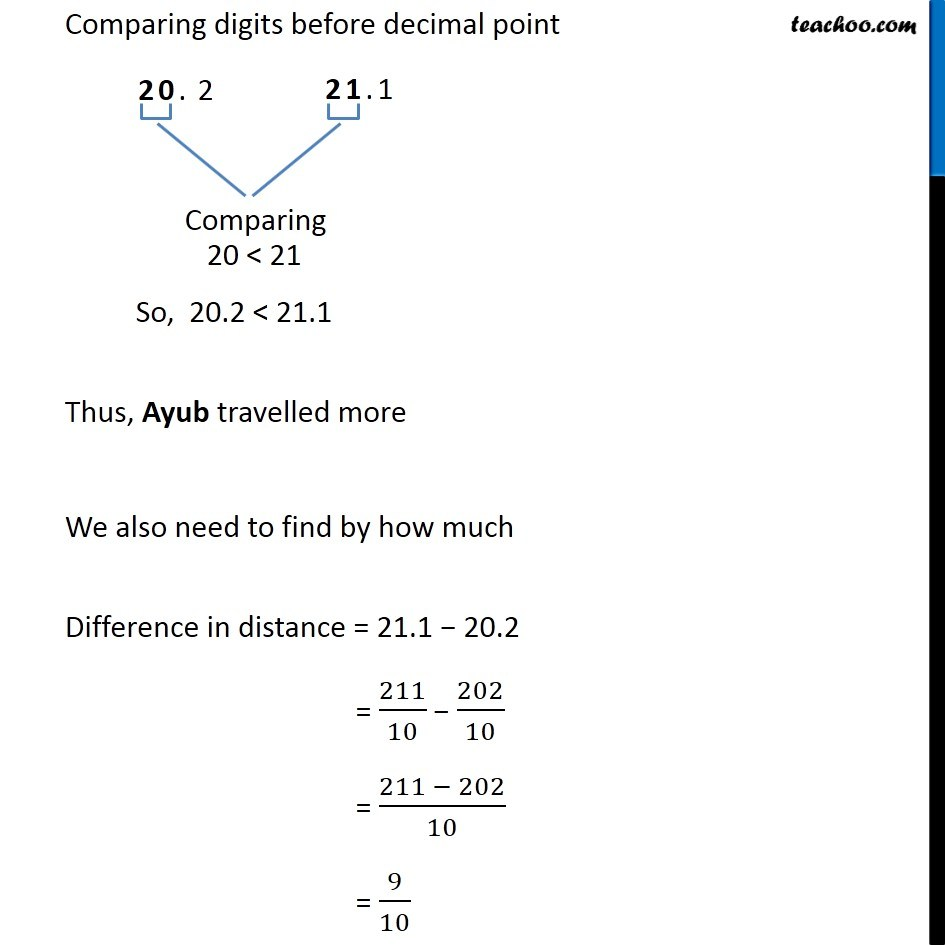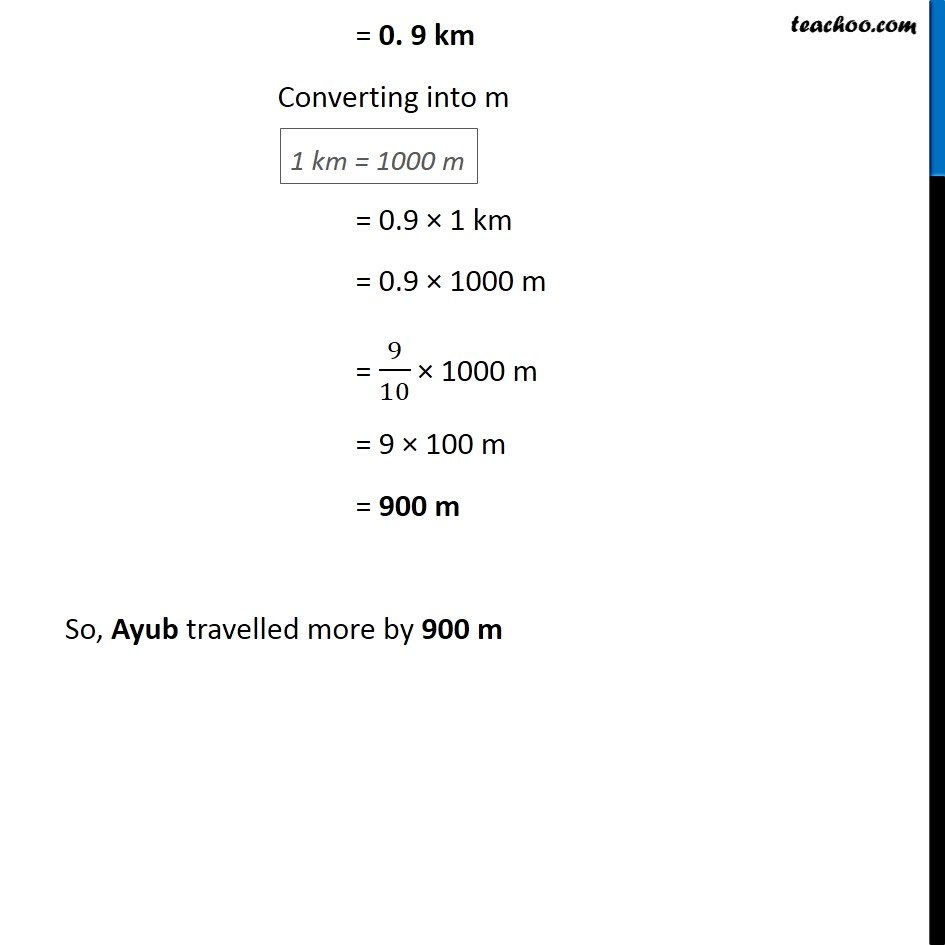Chapter 2 Class 7 Fractions and Decimals
Serial order wiseLearn in your speed, with individual attention - Teachoo Maths 1-on-1 Class

### Transcript

Question 7 Dinesh went from place A to place B and from there to place C. A is 7.5 km from B and B is 12.7 km from C . Ayub went from place A to place D and from there to place C. D is 9.3 km from A and C is 11.8 km from D. Who travelled more and by how much?Dinesh Dinesh went from A to B to C Total distance = AB + BC = 7.5 + 12.7 = 75/10 + 127/10 Ayub Ayub went from A to D to C Total distance = AD + DC = 9.3 + 11.8 = 93/10 + 118/10 = ((75 + 127))/10 = ((127 + 75))/10 = 202/10 = 20.2 km = ((93 + 118))/10 = ((118 +93))/10 = 211/10 = 21.1 km We need to find who travelled more So, we need to find greater of 20.2 & 21.1 Comparing digits before decimal point 20 < 21 So, 20.2 < 21.1 Thus, Ayub travelled more We also need to find by how much Difference in distance = 21.1 − 20.2 = 211/10 − 202/10 = (211 − 202)/10 = 9/10 = 0. 9 km Converting into m = 0.9 × 1 km = 0.9 × 1000 m = 9/10 × 1000 m = 9 × 100 m = 900 m So, Ayub travelled more by 900 m